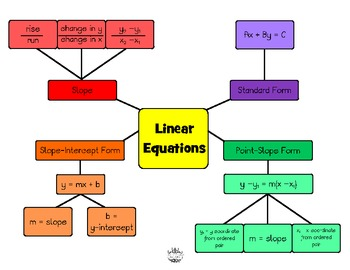# Ms. Minner's Classroom

A Louisville Middle School Classroom

The direction and steepness of a line is called slope. Slope on a graph represents the rate of change between data points. Slope (m), rate of change, and constant of proportionality (k) are synonyms with slight variations in meaning. The letter m is used to represent slope. There are four types of slope: positive (increasing), negative (decreasing), zero, and undefined.Linear means “straight line.” A linear equation is an equation whose solution when graphed forms a straight line. Linear equations can be either proportional (y=kx) in which k represents the constant of proportionality (rate of change), or they can be nonproportional (y=mx+b) in which m represents the slope (rate of change) and b represents the point at which the line intersects the y-axis.  This point of intersection with the y-axis is called the y-intercept. If you know the slope and y-intercept of a line, it is easy to write an equation that represents the line in slope-intercept form (y=mx+b). Given an equation in slope-intercept form, you can also use the y-intercept and slope to graph the line. Remember, that in order for a relationship to be proportional, the origin (0, 0) must be one solution of the equation. Any point on the graphed line representing a linear equation is a solution of that equation. Points on the line represent ordered pairs that will make the equation true.Save the Zogs-Linear Equations